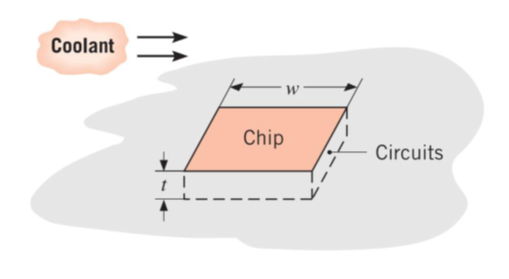## Cooling a CPU

A square silicon chip with a thermal conductivity of 150 W/m∙K is of width w = 7 mm on a side and of thickness t = 2 mm. The chip is mounted in a substrate such that its side and back surfaces are insulated, while the front surface is exposed to a coolant. If 60 W are being dissipated in circuits mounted to the back surface of the chip, what is the steady-state temperature difference between back and front surfaces?Hint
Fourier's Law of Conduction:
$$P=\dot{Q}=kA\frac{\Delta T}{t}$$$where $$P$$ is power, $$\dot{Q}$$ is the rate of heat transfer, $$k$$ is the thermal conductivity, $$A$$ is the surface area perpendicular to the heat transfer direction, $$\Delta T$$ is the change in temperature, and $$t$$ is the thickness. Hint 2 Because the silicon chip is a square profile: $$A=w^2$$$
where $$w$$ is the width.
Assumptions : (1) Steady state conditions, (2) Constant properties, (3) Uniform heat dissipation, (4) Negligible heat loss from back and sides, (5) One-dimensional conduction chip.

All the electrical power dissipated at the chip's back surface is transferred by conduction. From Fourier's Law of Conduction:
$$P=\dot{Q}=kA\frac{\Delta T}{t}$$$where $$P$$ is power, $$\dot{Q}$$ is the rate of heat transfer, $$k$$ is the thermal conductivity, $$A$$ is the surface area perpendicular to the heat transfer direction, $$\Delta T$$ is the change in temperature, and $$t$$ is the thickness. Rearranging the formula to solve for the temperature difference: $$\Delta T=\frac{t\cdot P}{kw^{2}}=\frac{0.002m\cdot 60W}{150W/m\cdot K(0.007m)^{2}}=16.33K$$$
16.33 K